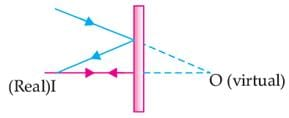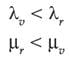# Assertion & Reason Test: Light- Reflection & Refraction - 1

## 15 Questions MCQ Test Online MCQ Tests for Class 10 | Assertion & Reason Test: Light- Reflection & Refraction - 1

Description
Attempt Assertion & Reason Test: Light- Reflection & Refraction - 1 | 15 questions in 30 minutes | Mock test for Class 10 preparation | Free important questions MCQ to study Online MCQ Tests for Class 10 for Class 10 Exam | Download free PDF with solutions
QUESTION: 1

### Directions: In the following questions, a statement of assertion (A) is followed by a statement of reason (R). Mark the correct choice as:Assertion (A): Plane mirror may form real image.Reason (R): Plane mirror forms virtual image, if object is real.

Solution: Plane mirror may form real image, if object is virtual.QUESTION: 2

### Directions: In the following questions, a statement of assertion (A) is followed by a statement of reason (R). Mark the correct choice as:Assertion (A): The image formed by a concave mirror is certainly real if the object is virtual.Reason (R): The image formed by a concave mirror is certainly virtual if the object is real.

Solution: The image of real object may be real in case of concave mirror.
QUESTION: 3

### Directions: In the following questions, a statement of assertion (A) is followed by a statement of reason (R). Mark the correct choice as:Assertion (A): Refractive index of glass with respect to air is different for red light and violet light.Reason (R): Refractive index of a pair of media depends on the wavelength of light used.

Solution: Refractive index of any pair of media is inversely proportional to wavelength of light. Hence,where, λv and λr are the wavelengths of violet and red light. μv and μr are refractive index of violet and red light.

QUESTION: 4

Direction: In the following questions, a statement of assertion (A) is followed by a statement of reason (R). Mark the correct choice as:

Assertion : Keeping a point object fixed, if a plane mirror is moved, the image will also move.

Reason : In case of a plane mirror, distance of object and Its image is equal from any point on the mirror.

Solution: Assertion (A) is false but reason (R) is true.
QUESTION: 5

Direction: In the following questions, a statement of assertion (A) is followed by a statement of reason (R). Mark the correct choice as:

Assertion : If a spherical mirror is dipped in water, its focal length remains unchanged.

Reason : A laser light is focused by a converging lens. There will be a significant chromatic aberration.

Solution: Assertion (A) is true but reason (R) is false.
QUESTION: 6

Direction: In the following questions, a statement of assertion (A) is followed by a statement of reason (R). Mark the correct choice as:

Assertion : Plane mirror may form real image.

Reason : Plane mirror forms virtual image, if objects is real.

Solution: Both assertion (A) and reason (R) are true but reason (R) is not the correct explanation of assertion (A). Plane mirror forms virtual image of real object and real image of virtual object.
QUESTION: 7

Direction: In the following questions, a statement of assertion (A) is followed by a statement of reason (R). Mark the correct choice as:

Assertion : Light travels faster in glass than in air.

Reason : Glass is denser than air.

Solution: Assertion (A) is false but reason (R) is true.
QUESTION: 8

Direction: In the following questions, a statement of assertion (A) is followed by a statement of reason (R). Mark the correct choice as:

Assertion : The height of an object is always considered positive.

Reason : An object is always placed above the principal axis in this upward direction.

Solution: Both assertion (A) and reason (R) are true and reason (R) is the correct explanation of assertion (A).
QUESTION: 9

Direction: In the following questions, a statement of assertion (A) is followed by a statement of reason (R). Mark the correct choice as:

Assertion : When a concave mirror is held under water, its focal length will increase.

Reason : The focal length of a concave mirror is independent of the medium in which it is placed.

Solution: Assertion (A) is false but reason (R) is true.
QUESTION: 10

Direction: In the following questions, a statement of assertion (A) is followed by a statement of reason (R). Mark the correct choice as:

Assertion : A convex mirror is used as a driver’s mirror.

Reason : Because convex mirror’s field of view is large and images formed are virtual, erect and diminished.

Solution: Both assertion (A) and reason (R) are true and reason (R) is the correct explanation of assertion (A).
QUESTION: 11

Direction: In the following questions, a statement of assertion (A) is followed by a statement of reason (R). Mark the correct choice as:

Assertion : A virtual image cannot be photographed.

Reason : Only real objects are photographed.

Solution: Assertion (A) is true but reason (R) is false. An image is a plane mirror is virtual and it can be photographed.
QUESTION: 12

Direction: In the following questions, a statement of assertion (A) is followed by a statement of reason (R). Mark the correct choice as:

Assertion : Mirror formula can be applied to a plane mirror.

Reason : A plane mirror is a spherical mirror of infinite focal length.

Solution: Both assertion (A) and reason (R) are true and reason (R) is the correct explanation of assertion (A).
QUESTION: 13

Direction: In the following questions, a statement of assertion (A) is followed by a statement of reason (R). Mark the correct choice as:

Assertion : For observing traffic at back, the driver mirror is convex mirror.

Reason : A convex mirror has much larger field of view than a plane mirror.

Solution: Both assertion (A) and reason (R) are true and reason (R) is the correct explanation of assertion (A).
QUESTION: 14

Direction: In the following questions, a statement of assertion (A) is followed by a statement of reason (R). Mark the correct choice as:

Assertion : When the object moves with a velocity 2 m/s, its image in the plane mirror moves with a velocity of 4 m/s.

Reason : The image formed by a plane mirror is as far behind the mirror as the object is in front of it.

Solution: Both assertion (A) and reason (R) are true and reason (R) is the correct explanation of assertion (A).
QUESTION: 15

Direction: In the following questions, a statement of assertion (A) is followed by a statement of reason (R). Mark the correct choice as:

Assertion : The small object, to be seen in a microscope, is kept within the two foci of its objective.

Reason : In this case, the image formed by the objective is nearer to the eyepiece.

Solution: Assertion (A) is true but reason (R) is false. Object is placed between F and 2F of objective lens.Use Code STAYHOME200 and get INR 200 additional OFF Use Coupon Code